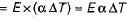Courses

# Test: Tension, Compression & Shear - 1

## 10 Questions MCQ Test Topicwise Question Bank for Mechanical Engineering | Test: Tension, Compression & Shear - 1

Description
This mock test of Test: Tension, Compression & Shear - 1 for Mechanical Engineering helps you for every Mechanical Engineering entrance exam. This contains 10 Multiple Choice Questions for Mechanical Engineering Test: Tension, Compression & Shear - 1 (mcq) to study with solutions a complete question bank. The solved questions answers in this Test: Tension, Compression & Shear - 1 quiz give you a good mix of easy questions and tough questions. Mechanical Engineering students definitely take this Test: Tension, Compression & Shear - 1 exercise for a better result in the exam. You can find other Test: Tension, Compression & Shear - 1 extra questions, long questions & short questions for Mechanical Engineering on EduRev as well by searching above.
QUESTION: 1

### A steel bar of rectangular cross-section (20 x 50 mm) carries a tensile load P and is attached to a support by means of a round pin of diameter 20 mm. The allowable stresses for the bar in tension and the pin in shear are σallow = 150 MPa and τallow = 75 MPa, respectively. What is the maximum permissible value of the load P?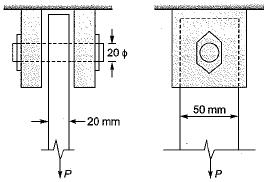Solution:

= 20 x (50 - 20) = 600 mm2
∴ P1 (based on tensile stress)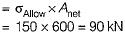Net area for shearing load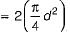∴ P2 (based on shearing stress)
= 75 x 2 x 0.785 x 400 = 47.1 kN
So, permissible value of Pis 47 kN.

QUESTION: 2

Solution:
QUESTION: 3

### A rod of variable cross-section (areas A1 and A2) fixed at one end is subjected to an axial force Pas shown below.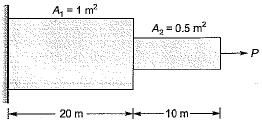If the allowable normal stress in tension is 100 MPa, then the maximum allowable load (neglecting stress concentration) is

Solution: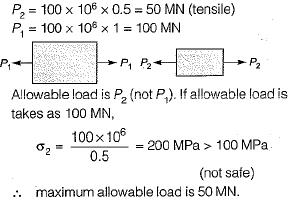QUESTION: 4

A straight bar having a constant cross-sectional area A, length L and weight W is hanging vertically. If E is the Young’s modulus of the material, total increase in length of the bar due to its own weight only is

Solution: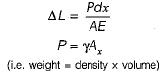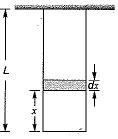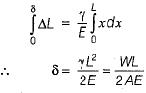QUESTION: 5

In a composite system subjected to temperature rise and with ends constrained to remain together, the component having lower value of coefficient of linear expansion will experience a

Solution:

With ends constrained to remains together, the component with higher coefficient of expansion will try to pull the component having low value of expansion coefficients. Apparently there will be tensile force in the component of lower α and a compressive force in the component of higher α as the former will try to restrict the later from expanding more.

QUESTION: 6

All of the following statements are correct, except.

Solution:

Each member of a composite bar is subjected to same strain.

QUESTION: 7

A steel rod passes through a brass tube which is closed by thin rigid washers and fastened by nuts screwed to the rod. Which type of stress will be induced in the tube when the nut is tightened on the bolt?

Solution:

When nut is tightened on the bolt, rod is subjected to tensile stress and brass tube is subjected to compressive state of stress.

QUESTION: 8

Two wires of different materials but of same diameter are connected end to end and a force is applied which stretches them by 1 cm. The two wires will have the

Solution: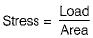Load and area remains same for both cases, so stresses are same.
Stress = E x strain
E is different for both materials, so strains are different.

QUESTION: 9

A steel wire ( E = 200 GPa) of sectional area 10 x 10-5 m2 is to be extended to twice its initial length. To accomplish this task, the two ends of the wire should be pulled by a force of

Solution: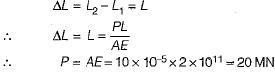QUESTION: 10

A steel rod of length 2 and diameter d, fixed at both ends is uniformly heated to a temperature rise of ΔT. The Young’s modulus is E and the coefficient of linear expansion is α. The thermal stress in the rod is

Solution:

Thermal stress = E x Thermal strain